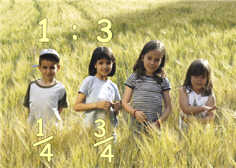Definition of

# RatioA ratio shows the relative sizes of two or more values.

Ratios can be shown in different ways:
• using the ":" to separate example values
• using the "/" to separate one value from the total
• as a decimal, after dividing one value by the total
• as a percentage, after dividing one value by the total

Example: if there is 1 boy and 3 girls you could write the ratio as:

1:3 (for every one boy there are 3 girls)
1/4 are boys and 3/4 are girls
0.25 are boys (by dividing 1 by 4)
25% are boys (0.25 as a percentage)

../numbers/images/ratio.js
See: Scale